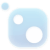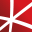# Top 20 NuGet probability Packages

Math.NET Numerics is the numerical foundation of the Math.NET project, aiming to provide methods and algorithms for numerical computations in science, engineering and every day use. Supports .NET 5.0 or higher, .NET Standard 2.0 and .NET Framework 4.6.1 or higher, on Windows, Linux and Mac. This pac...
Math.NET Numerics is the numerical foundation of the Math.NET project, aiming to provide methods and algorithms for numerical computations in science, engineering and every day use. Supports .NET 5.0 or higher, .NET Standard 2.0 and .NET Framework 4.6.1 or higher, on Windows, Linux and Mac.
F# Modules for Math.NET Numerics, the numerical foundation of the Math.NET project, aiming to provide methods and algorithms for numerical computations in science, engineering and every day use. Supports .NET 5.0 or higher, .NET Standard 2.0 and .NET Framework 4.6.1 or higher, on Windows, Linux and ...
The GPU-accelerated version of package CenterSpace.NMath.Stats. With a few minor exceptions, such as optional GPU configuration settings, the API is identical between CenterSpace.NMath.Stats.Premium and CenterSpace.NMath.Stats.
Classes for data manipulation and statistical computation, including descriptive statistics, probability distributions, combinatorial functions, multiple linear regression, hypothesis testing, analysis of variance, multivariate statistics, partial least squares, and nonnegative matrix factorization.
The Extreme Optimization Numerical Libraries for .NET are a set of libraries for numerical computing and data analysis. This is the main package that contains all the core functionality. For optimal performance, we strongly recommend also referencing one of the native packages based on Intel's Mat...## Math.NET Numerics - MKL Native Provider for Windows (x64)

Intel oneAPI MKL native libraries for Math.NET Numerics on Windows.
F# Modules for Math.NET Numerics, the numerical foundation of the Math.NET project, aiming to provide methods and algorithms for numerical computations in science, engineering and every day use. Supports .NET 5.0 or higher, .NET Standard 2.0 and .NET Framework 4.6.1 or higher, on Windows, Linux and ...
Foundational classes for financial, engineering, and scientific applications, including complex number classes, general vector and matrix classes, structured sparse matrix classes and factorizations, general sparse matrix classes and factorizations, general matrix decompositions, least squares solut...## Math.NET Numerics - MKL Native Provider for Windows (x64 and x86)

Intel oneAPI MKL native libraries for Math.NET Numerics on Windows.## Math.NET Numerics - MKL Native Provider for Windows (x86)

Intel oneAPI MKL native libraries for Math.NET Numerics on Windows.## mXparser – Math Expressions Parser / Formula Evaluator library for JAVA Android C# .NET/MONO (.NET Framework, .NET Core, .NET Standard, .NET PCL, Xamarin.Android, Xamarin.iOS) CLS compliant

mXparser is a super easy, rich, fast and highly flexible math expression parser library (parser and evaluator of mathematical expressions / formulas provided as plain text / string). Software delivers easy to use API for JAVA, Android and C# .NET/MONO (Common Language Specification compliant: F#, Vi...
Class library implementing advanced mathematical algorithms, transforms, and time series manipulations. Implementations favour simplicity and correctness.
Foundational classes for financial, engineering, and scientific applications, including complex number classes, general vector and matrix classes, structured sparse matrix classes and factorizations, general sparse matrix classes and factorizations, general matrix decompositions, least squares solut...
Foundational classes for financial, engineering, and scientific applications, including complex number classes, general vector and matrix classes, structured sparse matrix classes and factorizations, general sparse matrix classes and factorizations, general matrix decompositions, least squares solut...## NMath - Standard Library - Windows and Linux - x64

Foundational classes for financial, engineering, and scientific applications, including complex number classes, general vector and matrix classes, structured sparse matrix classes and factorizations, general sparse matrix classes and factorizations, general matrix decompositions, least squares solut...## NMath - Standard Library - Windows - x86 and x64

Foundational classes for financial, engineering, and scientific applications, including complex number classes, general vector and matrix classes, structured sparse matrix classes and factorizations, general sparse matrix classes and factorizations, general matrix decompositions, least squares solut...
Foundational classes for financial, engineering, and scientific applications, including complex number classes, general vector and matrix classes, structured sparse matrix classes and factorizations, general sparse matrix classes and factorizations, general matrix decompositions, least squares solut...
The Extreme Optimization Numerical Libraries for .NET are a set of libraries for numerical computing and data analysis. This package contains the single-precision mixed-mode native provider. This is the recommended native provider for the classic .NET Framework on Windows. Supports .NET Framework ...
The Extreme Optimization Numerical Libraries for .NET are a set of libraries for numerical computing and data analysis. This package contains the single-precision mixed-mode native provider. This is the recommended native provider for the classic .NET Framework on Windows. Supports .NET Framework ...